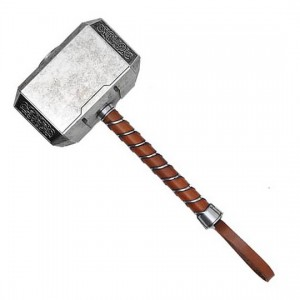# teaching machines

## SCHUHAJ – I Madeup Life

May 9, 2015 by . Filed under cs455, postmortems, spring 2015.

I looked at what had existed so far, and upon seeing Corey’s thought on making a double helix, I wanted to make one.

First I needed to make a spiral,

for i to 5
for t in 0,30..360
x = 10 * cos t
y = 10 * sin t
z = 0.1 * (360 * i + t)
moveto x, y, z
end
end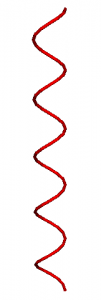Then I needed to be able to reverse down that same spiral to the beginning to start the second spiral.

for j in 4,3..0
for s in 360,330..0
x = 10 * cos s
y = 10 * sin s
z = 0.1 * (360 * j + s)
moveto x, y, z
end
end

Then I jumped over and made the second spiral.

for i through 4
for t in 0,30..360
x = 10 * cos (t + 180)
y = 10 * sin (t + 180)
z = 0.1 * (360 * i + t)
moveto x, y, z
end
end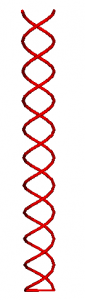I didn’t bother figuring out the reversing of this spiral, realizing I was going to alter my approach.

I knew I was going to be drawing at least some of the lines twice, so I altered the approach to a four step process:

Draw part of the first helix.  Jump across to the other helix.  Draw part of the second helix.  Jump across to the other helix.

helix = 0
hc = 180
d = 10

for i through 4
for t in 0,30..360
if helix == 0
x = d * cos t
y = d * sin t
z = 0.1 * (360 * i + t)
moveto x, y, z
x = d * cos (t+hc)
y = d * sin (t+hc)
moveto x, y, z
helix = 1
else
x = d * cos (t + hc)
y = d * sin (t + hc)
z = 0.1 * (360 * i + t)
moveto x, y, z
x = d * cos t
y = d * sin t
moveto x, y, z
helix = 0
end
end
end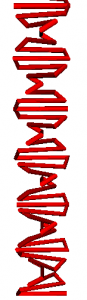Once I completed that, I was at the top, and just needed to make my way back down while drawing the gaps in each helix, only duplicating the jumps across the helixes.

BOOM!

Final code:

helix = 0
hc = 180
d = 10

for i through 4
for t in 0,30..360
if helix == 0
x = d * cos t
y = d * sin t
z = 0.1 * (360 * i + t)
moveto x, y, z
x = d * cos (t+hc)
y = d * sin (t+hc)
moveto x, y, z
helix = 1
else
x = d * cos (t + hc)
y = d * sin (t + hc)
z = 0.1 * (360 * i + t)
moveto x, y, z
x = d * cos t
y = d * sin t
moveto x, y, z
helix = 0
end
end
end

helix = 0
for i in 4,3..0
for t in 360,330..0
if helix == 0
x = d * cos t
y = d * sin t
z = 0.1 * (360 * i + t)
moveto x, y, z
x = d * cos (t+hc)
y = d * sin (t+hc)
moveto x, y, z
helix = 1
else
x = d * cos (t + hc)
y = d * sin (t + hc)
z = 0.1 * (360 * i + t)
moveto x, y, z
x = d * cos t
y = d * sin t
moveto x, y, z
helix = 0
end
end
end

nsides = 10
tube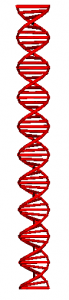The only hurdles I faced were my limitations in geometry.  I had to walk away from the computer many times…

The only bugs I faced were:

Hitting “fit” button multiple times would make it switch between actually fitting, a blank screen, and a screen with strange dark pixels

Adding comments will make the code not work.  (Particularly after an else statement and on its own line.)

I kept playing with the program making stupid things…

The useless hammer: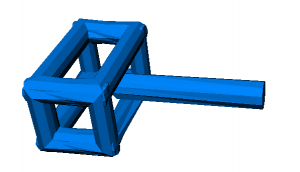Worthwhile hammer: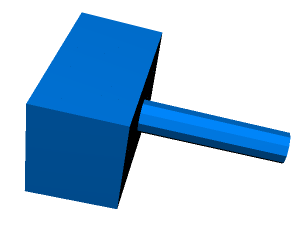Mjolnir: